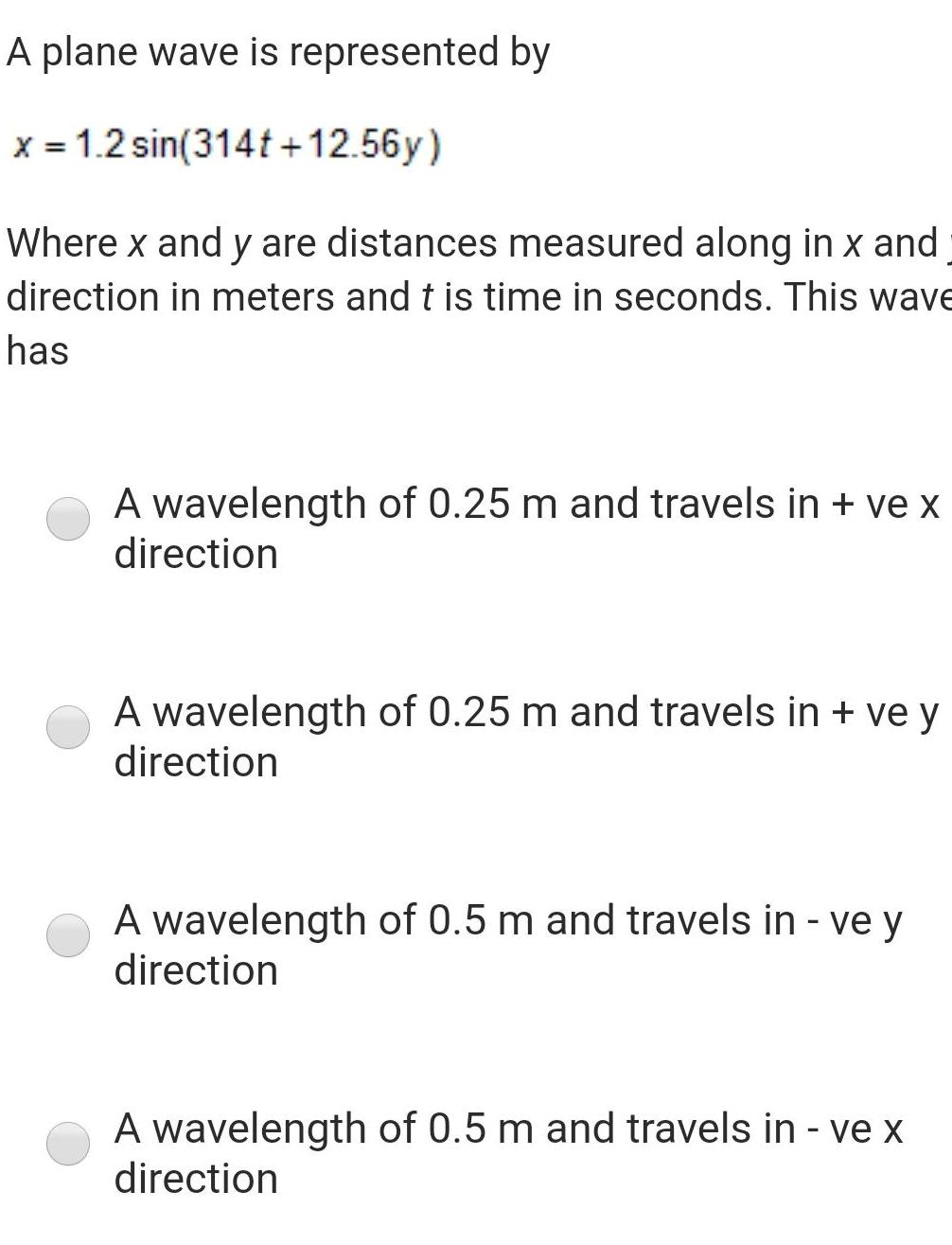Question:

# A plane wave is represented by x 1 2 sin 314t 12 56y Where x

Last updated: 6/8/2023A plane wave is represented by x 1 2 sin 314t 12 56y Where x and y are distances measured along in x and direction in meters and t is time in seconds This wave has A wavelength of 0 25 m and travels in ve x direction A wavelength of 0 25 m and travels in ve y direction A wavelength of 0 5 m and travels in ve y direction A wavelength of 0 5 m and travels in ve x direction